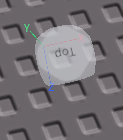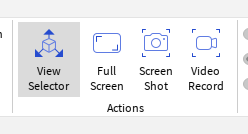# Did I get the angle correctly?

I tried getting the angle between Part A and Part B, Did I do it correctly?
The angle it outputted was 48 degrees

Here is the code:

``````local Key1 = game.Workspace.PartA
local Key2 = game.Workspace.PartB

local PosDiff = Key1.Position - Key2.Position

local XDiff = math.abs(PosDiff.X) -- 61.71
local ZDiff = math.abs(PosDiff.Z) -- 69.50
-- Hypothenus is 93, I didnt need it in the ATan formula.

print(math.deg(math.atan2(ZDiff, XDiff)) -- Output: 48

``````

Here is a visual representation I did based off what I think is happening behind the scenes:
did I visualize it correctly?

2 Likes

1 Like

Hi againI only think that solution would work in the first quadrant. The cool thing about `atan2` is that it works in all 4 quadrants, but uses two numbers vs normal `atan`'s single number. However it needs to know the signs of the input numbers (+/-), so taking the absolute value of the position difference removes important information that it needs.

Try it all around the circle, IMO it should only work without the `abs`. Other than that yeah it looks right, I think you even have the order of parameters to `atan2` right which can be a bit confusing.

1 Like

Hey, I removed the absolute value function.

Now the angle dont seem to fit in/match up, How is it being calculated?

The output is 132 degrees, shouldn’t it be 48 degrees: the way I mapped it out visually?

Code:

``````local Key1 = game.Workspace.Key1
local Key2 = game.Workspace.Key2

local PosDiff = Key1.Position - Key2.Position
local XDiff = PosDiff.X -- 69.50
local ZDiff = PosDiff.Z -- 69.50

print(math.deg(math.atan2(ZDiff, XDiff))) -- Output 132
``````
1 Like

Yeah, it definitely looks like 48 should be right. Can you try negating the 1st parameter to `atan2`? I.e. `math.atan2(-ZDiff, XDiff)`. I think it’s the opposite of a normal coordinate system because Roblox’s front vector is (0, 0, -1) and not (0, 0, 1). You can see the XYZ axes in Studio using the View Selector (it’s really hard to see in this screenshot).2 Likes

I tried negating the 1st parameter, it didnt work, then I tried the negatiting the X(Second Paramater) and it worked. The angle now displays properly,Thank you for assisiting.

1 Like